# Awesome ChatGPT prompts for spreadsheet usersWritten by
Marcos Murata

ChatGPT has become the go-to tool for hundreds of millions of Internet users over the past few months. What’s more, ChatGPT’s unparalleled rise to fame has spawned a surge in searches for all sorts of artificial-intelligence tools.

More and more users are looking for tools that can help them tackle their daily tasks more efficiently. Amid this AI frenzy, spreadsheet users are also looking for some help with their data manipulation and analysis.

Although some AI tools such as Ajelix and Excelformulabot are already capitalizing on this trend, it seems that spreadsheet users still haven’t found a reliable platform that could be their favorite assistant.

Meanwhile, ChatGPT itself could give a helping hand to anyone who is struggling with spreadsheets. While it may struggle to create more complex formulas, this AI-powered tool can help users perform less complicated calculations. All you need to do is ask the right questions and use the right prompts.

If you don’t know how to take advantage of this powerful AI tool, check out these awesome ChatGPT prompts that can be of great help to all spreadsheet users.

### ChatGPT prompts for Google Sheets

• How can I sum values from two cells in Google Sheets?
• How can I sum only numbers greater than 10 in Google Sheets?
• How can I calculate the average of three cells in Google Sheets?
• How can I find the highest number in a range in Google Sheets?
• How can I find the lowest number in a range in Google Sheets?
• How can I divide numbers in Google Sheets?
• How can I multiply values from two cells in Google Sheets?
• How can I subtract values from two cells in Google Sheets?
• How can I count how many cells within a certain range contain a specific value in Google Sheets?
• How can I sum the values in cells A1 and B1, and multiply the total to the value in cell C1 in Google Sheets?
• How can I calculate percentages in Google Sheets?
• How can I filter data from a range of cells in Google Sheets?
• How can I find the number of days between two dates in Google Sheets?
• How can I count how many unique values there are in a range of cells in Google Sheets?
• How can I create a pie chart in Google Sheets?
• How can I create a bar chart in Google Sheets?
• How can I create a scatter plot in Google Sheets?
• Write a formula in Google Sheets to find the highest number from all the values in column A, from row 1 to row 20.
• Write a formula in Google Sheets to calculate the average of all the values in column A, from row 1 to row 20.
• Write a formula to concatenate cell A1, cell B1 and cell C1 in Google Sheets.
• Write a formula to calculate the number of days between cells A1 and B1.
• Write a formula to count the number of occurrences of a certain value within a range in Google Sheets.

### Testing ChatGPT prompts for Google Sheets

Now that we’ve listed some useful prompts, we can put them to the test. We will use ChatGPT  to create a spreadsheet from scratch and perform some basic calculations.

Let’s start by creating some dummy data for our spreadsheet.

Create dummy data for a spreadsheet with five columns: Name, Age, Nationality, Email, Occupation, and Salary. Fill the table with 20 rows of data. Use these 5 nationalities randomly to fill the table: American, Canadian, German, Spanish and British.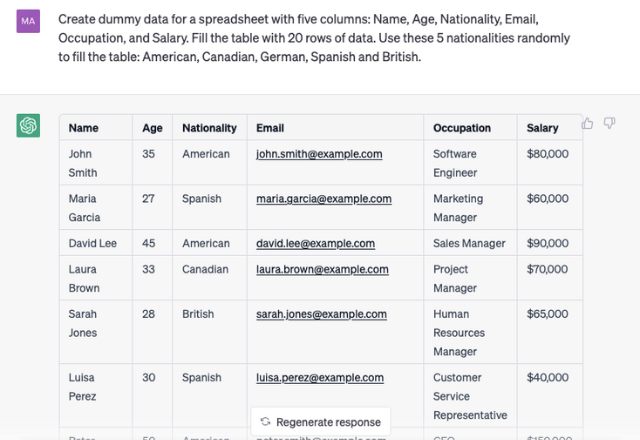After we copy and paste the data into a worksheet, our dummy dataset is ready for use.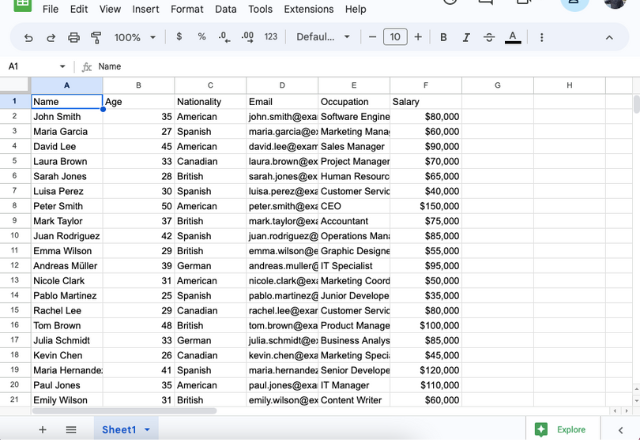First, let’s find the highest salary from the list by using the prompt below.

Write a formula in Google Sheets to find the highest number from all the values in column F, from row 2 to row 21.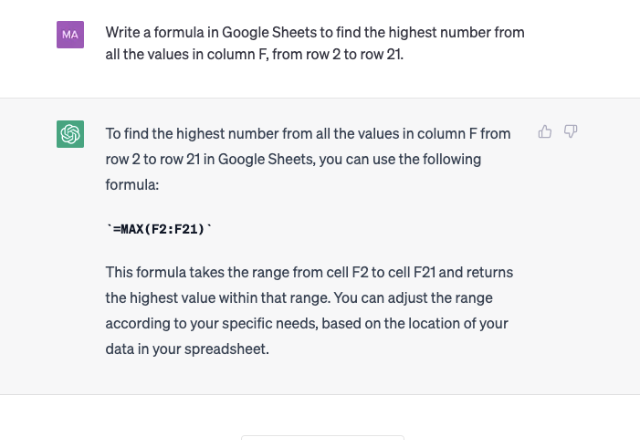Now we can use ChatGPT’s formula to find the highest salary.

=MAX(F2:F21)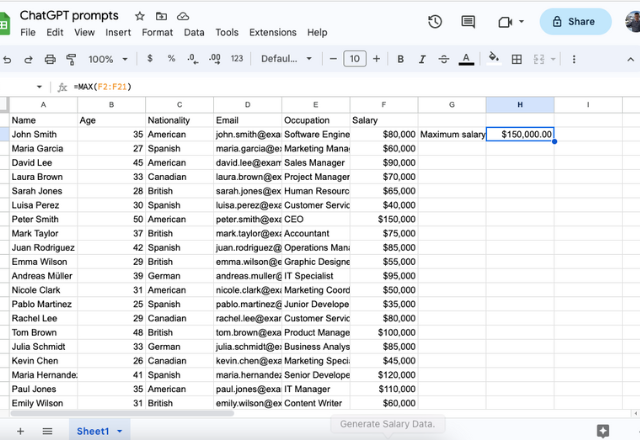We can now ask ChatGPT to create a formula to calculate the average salary.

Write a formula in Google Sheets to calculate the average of all the values in column F, from row 2 to row 21.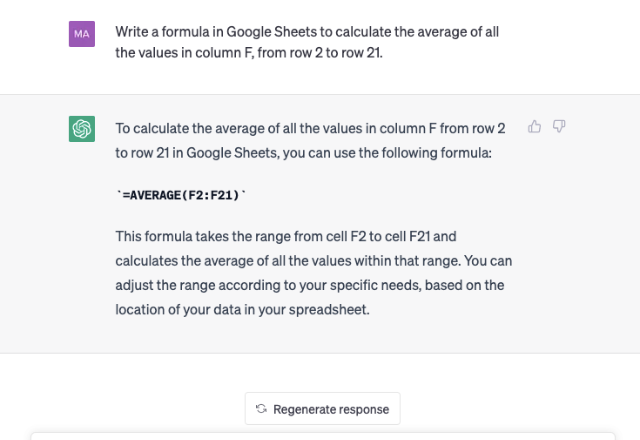Let’s add the formula to the worksheet now to calculate the average salary.

=AVERAGE(F2:F21)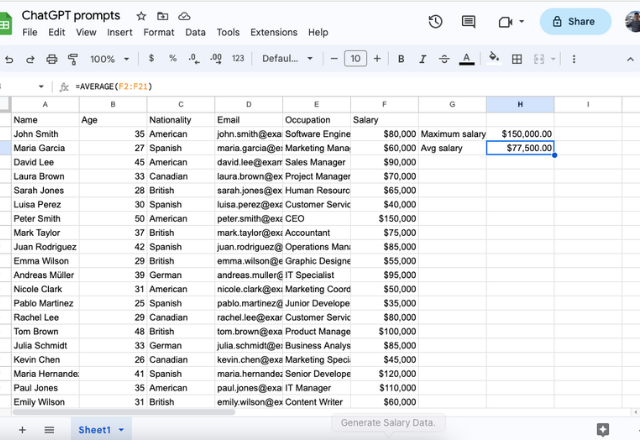Let’s say we want to calculate the average salary of all the American employees from the list. We can use the prompt below to ask ChatGPT for some help.

Write a formula in Google Sheets to calculate the average of all the values in column F, from row 2 to row 21, whose corresponding value in column C is ‘American’.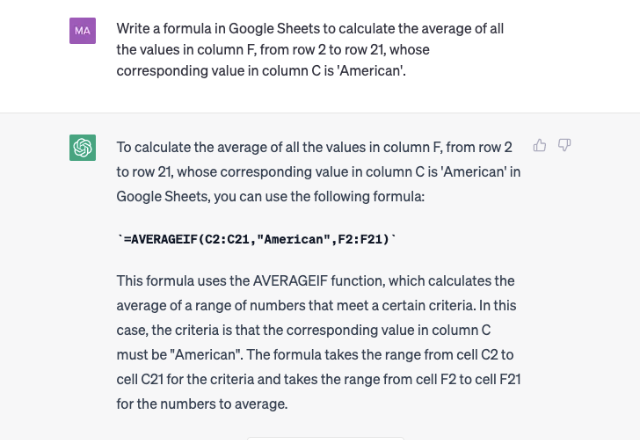Let’s test the formula now.

=AVERAGEIF(C2:C21,”American”,F2:F21)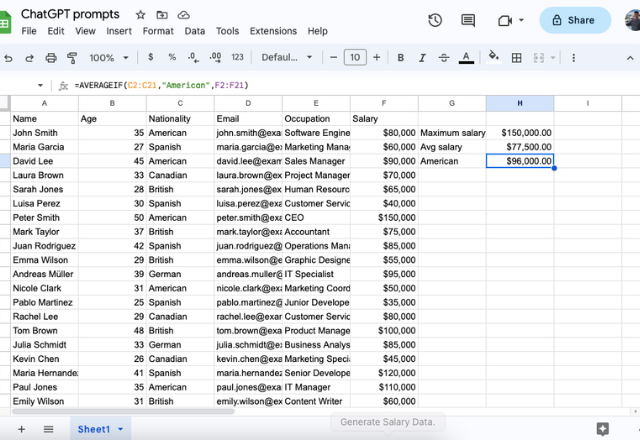There you go! Google Sheets has calculated the average salary of only the American employees. By feeding ChatGPT the right prompts, we could create some formulas to analyze data and perform basic calculations in Google Sheets.

You can use some of these awesome ChatGPT prompts to organize, manipulate and analyze your data.

However, ChatGPT still has some limitations when it comes to creating spreadsheet formulas.